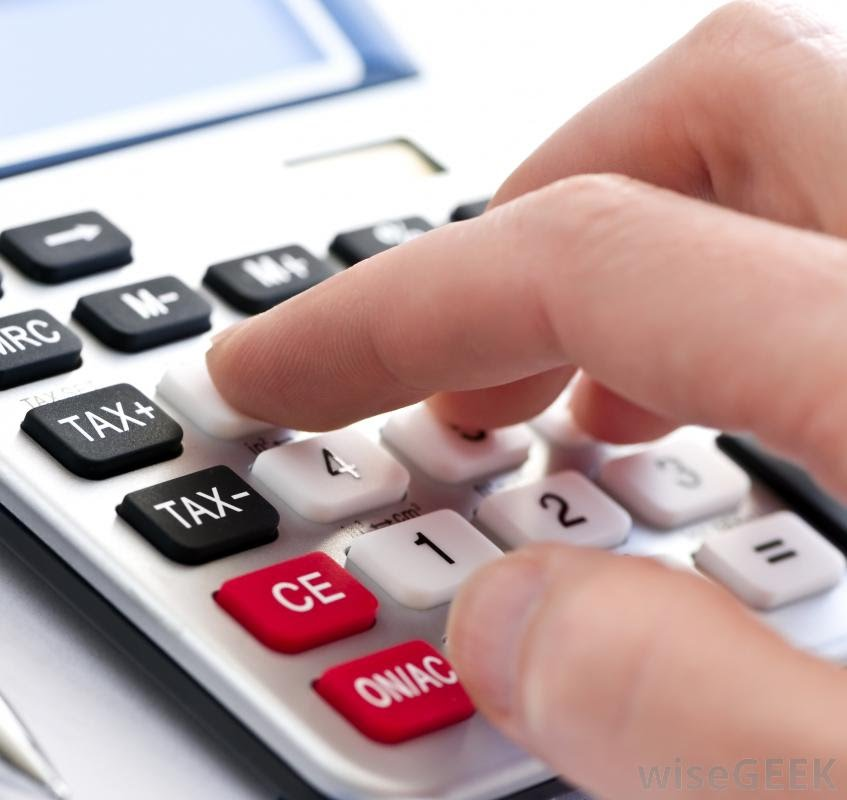digit puzzle

What 5 digit number am I thinking of.

It has two prime digits.

Digit 3 is the highest digit.
Digit 2 is lowest digit.
Digit 1 is higher than the sum of digits 4 and 5.
Digit 5 is half of digit 4.
Digit 1 is one smaller than digit 3.
Digit 5 is between digit 2 and digit 1
There are no duplicates and the digit 0 does not appear.

Do not forget that 1 is not prime.